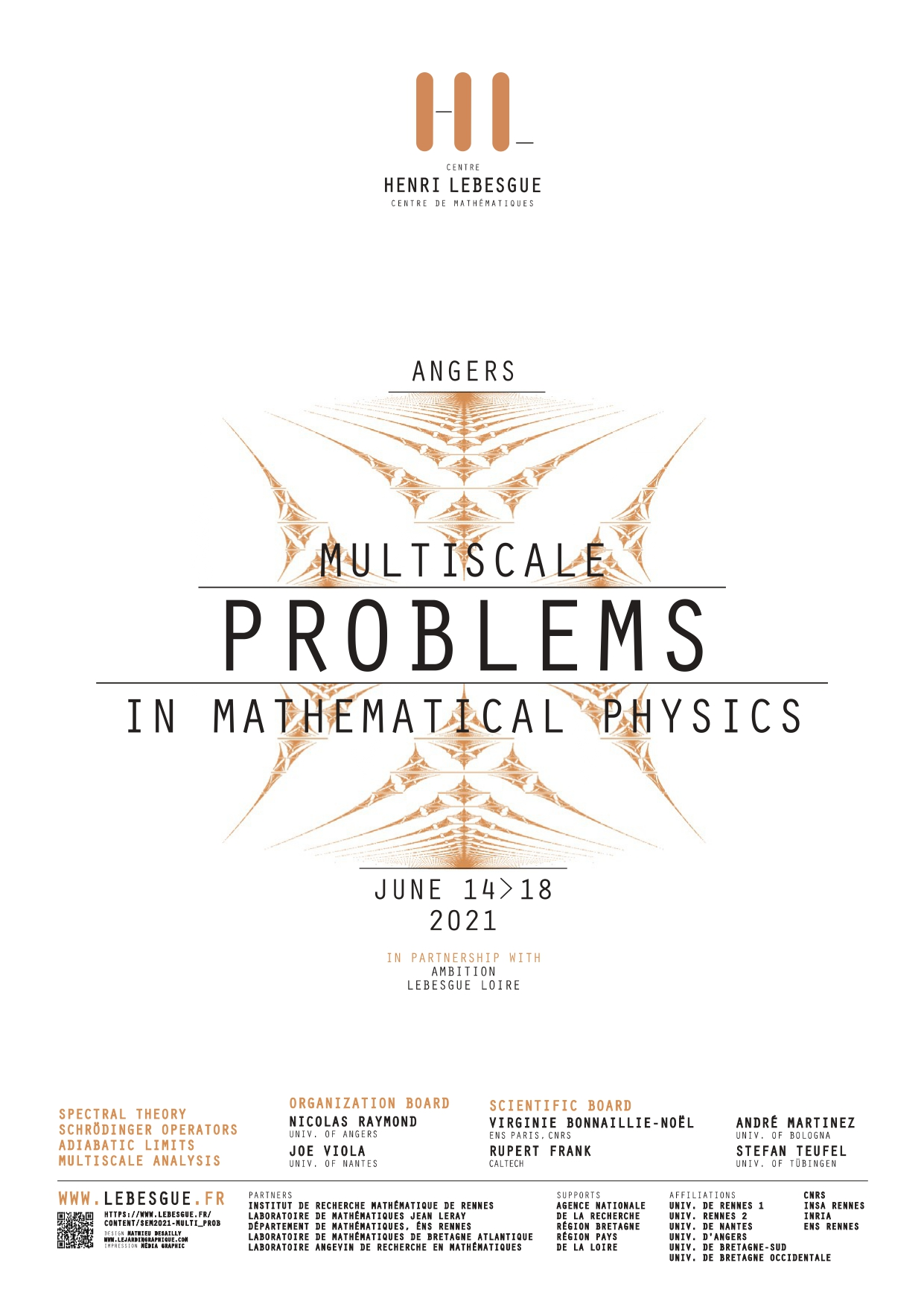placeholder

## Primary tabs

### Tuesday, June 15, 2021

All day
Conference - Multiscale problems in mathematical physics Jun 14, 2021 to Jun 16, 2021 read moreAngers, from june 14th to june 16th 2021

Organization board: Nicolas RAYMOND, Joe VIOLA

The aim of this workshop is to present the latest advances involving multiscale phenomena arising in Physics. This will allow for the interaction between different research communities and stimulate new investigations on a large array of recent problems, from dynamical problems to spectral theory.

### Speakers

Paul Alphonse (ENS Lyon)

Victor Arnaiz (Université Paris-Saclay)

Vincent Bruneau (Université de Bordeaux)

Mattia Cafasso (University of Angers)

Éric Cancès (École des Ponts Paristech)

Clotilde Fermanian Kammerer (University Paris-Est Creteil, CNRS)

Louis Garrigue (École des Ponts)

Frédéric Hérau (University of Nantes)

Jonas Lampart (Université de Bourgogne)

Loïc Le Treust (Université d'Aix Marseille)

Léo Morin (Université de Rennes 1)

Tiến-Tài Nguyễn (Université de Paris 13)

Van Hoi Nguyen (Université de Rennes 1)

Nicolas Rougerie (ENS Lyon)

Julien Sabin (École Polytechnique)

Nir Schwartz (Université Paris-Saclay)

Nikolay Tzevtkov (University of Cery-Pontoise)

 Before 01 01 02 03 04 05 06 07 08 09 10 11 12 13 14 15 16 17 18 19 20 21 22 23 Conference - Multiscale problems in mathematical physics Jun 15, 2021 I will discuss how the Dirac-Klein-Gordon system gives rise to the (cubic) nonlinear Dirac equation in the limit of strong coupling and large (field) mass. Joint work with Loic Le Treust, Simona Rota Nodari and Julien Sabin.     Conference - Multiscale problems in mathematical physics Jun 15, 2021 In this talk, we are interested in understanding the smoothing and the localizing properties of two classes of evolution equations. The first class is associated with the accretive quadratic operators (which are non-selfadjoint differential operators), for which partial hypoellipticity phenomena occur, which will be explained. The second class is associated with the fractional anisotropic Shubin operators (defined as the fractional power of differential operators), whose smoothing and localizing properties are subject to an uncertainty principle, as we will see. This a work partly joint with J. Bernier (LMJL).             Conference - Multiscale problems in mathematical physics Jun 15, 2021 Nous étudions la perturbation, par un obstacle, d'un hamiltonien magnétique en dimension 3. La présence de spectre continu nous amène à analyser les phénomènes de concentration spectrale en étudiant les singularités de la fonction de décalage spectrale. Suivant les conditions au bord de l'obstacle, nous montrons des phénomènes de concentration au dessus ou en dessous des niveaux de Landau. L'analyse asymptotique révèle la contribution de la capacité du projeté de l'obstacle dans la direction du champ magnétique. Travail réalisé en collaboration avec G. Raykov.     Conference - Multiscale problems in mathematical physics Jun 15, 2021 The linear instability study of the viscous Rayleigh-Taylor model in the neighborhood of a laminar solution $(\rho_0(x_3), \vec 0, p_0(x_3))$ of the incompressible Navier-Stokes system of equations in $\textbf{R}^3$ with a gravitational field $-g\vec e_3$ amounts to the study of the following ordinary differential equation of order 4: $\label{MainEq} -\lambda^2 [ \rho_0 k^2 \phi - (\rho_0 \phi')'] = \lambda \mu (\phi^{(4)} - 2k^2 \phi'' + k^4 \phi) - gk^2 \rho_0'\phi,$ where $\lambda$ is the desired growth rate in time, $k$ is the wave number transverse to the density profile. We first study the toy model $\rho_0(x_3) = \rho_+ 1_{\{x_3>0\}} + \rho_- 1_{\{x_3<0\}}$, with $\rho_{\pm}$ being positive constants (note that this profile is not smooth). We then derive the unique growth rate obtained for the toy model, hence generalize the well known inviscid Rayleigh-Taylor study between two immiscible fluids. Secondly, we consider two types of increasing profile, that are $\rho_0'$ being compactly supported and being positive everywhere. We prove that, contrary to the toy model, and in accordance with the results of Guo and Hwang, Helffer and Lafitte for the incompressible Euler system of equation with a gravitational field, there is an infinite sequence of non trivial solutions $(\lambda_n, \phi_n)$ of ([MainEq]), with $\lambda_n\rightarrow 0$ when $n\rightarrow +\infty$ and $\phi_n\in H^4(\textbf{R})$. This is my joint work with O. Lafitte.         Conference - Multiscale problems in mathematical physics Jun 15, 2021 We consider a model for two types (bath and tracers) of 2D quantum particles in a perpendicular (artificial) magnetic field. Interactions are short range and only inter-species, and we assume that the bath particles are fermions, all lying in the lowest Landau level of the magnetic field. Heuristic arguments then indicate that, if the tracers are strongly coupled to the bath, they effectively change their quantum statistics, from bosonic to fermionic or vice-versa. We rigorously compute the energy of a natural trial state, indeed exhibiting this phenomenon of statistics transmutation. The proof is based on (seemingly ?) new estimates for the characteristic polynomial of the Ginibre ensemble of random matrices. Joint work with Douglas Lundholm and Gaultier Lambert